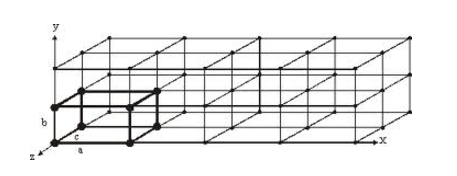# I'm in the space!

Probability Level 3There are $n$ distinct lattice points marked in the 3D space.

Find least possible value of $n$, such that we can always choose 2 points out of $n$ points (wherever they may be marked), such that there's at least one more lattice point on the segment joining them.

Details and assumptions:-

$\bullet$ In the 3D space, every point can be represented as coordinates $(x,y,z)$, where $x,y,z \in \mathbb{R}$

$\bullet$ Lattice points are points that have integer coordinates.

Easier version 2D

Harder version 5D

×

Problem Loading...

Note Loading...

Set Loading...